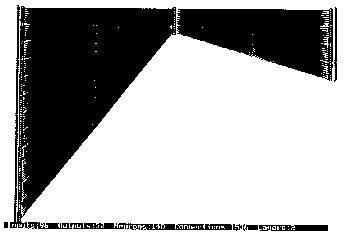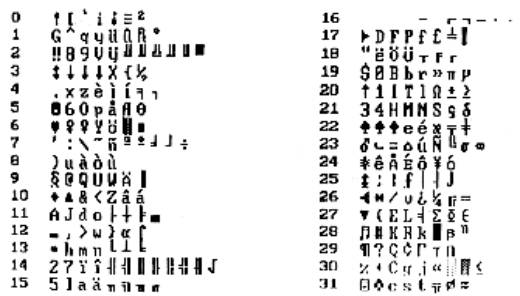## RENT A THINKER Home My Page Chat Tik-Tok 4        A Learning Algorithm For Self-Organized Multi-layered Neural Network

### 4.1       Abstract

Feed Forward type multilayered Neural Networks are very popular for their generalization and feature extraction property. However, the Feed Forward type network needs supervised learning to train the network initially. Self-organizing networks on the other hand are used in application of image classification, speech recognition, language translation etc. An algorithm is developed to train multilayered Feed Forward type Network in both supervised and unsupervised mode. Unsupervised learning is achieved by enhancing the maximum output and de clustering the crowded classes simultaneously. Proposed algorithm allows forcing any output to learn a desired class using supervised training like Back Propagation. The Network uses multilayered architecture and hence it classifies the features. Population of each class could be controlled with greater flexibility.

### 4.2       Introduction

Multilayered Feed Forward type Neural Networks (FFNN) are most popular and are often used with supervised learning algorithms like Back Propagation. These networks have excellent properties like generalization, feature extraction, mapping of nonlinear boundary etc. Once a FFNN is trained with a set of input-output, it cannot respond to new features/patterns. For example if FFNN is trained with speech of a person, it will not recognize the voices of others, until it is trained again. Also it needs precise knowledge of output during training operation. It is often seen that the multilayered network training could be accelerated by individually training the hidden layers in unsupervised mode. Complex networks are often hierarchical in nature and the individual network needs generalized and unsupervised training to organize and classify inputs. A general-purpose algorithm is designed to organize the data using unsupervised learning. The algorithm is similar to conventional Back Propagation technique, except the error function do not have any desired output. Desired output is replaced by combination of two vectors, (a) maximum output element and (b) average of occurrence of maximum output. The first vector used as excitatory and the second one as inhibitory element. This algorithm does not use the boundary convergence around the maxima like Kohonen’s algorithm. Hence the convergence of class boundaries need not be synchronized with the convergence of error.

### 4.3       Proposed Algorithm

Multilayered Feed Forward type networks are trained by minimizing the energy function of output error in the form of

E = ½ å (Yk – Dk) 2 ….(1)

Where Dk is the desired output and Yk is the actual output of the network.

Any network element Wij is modified in proportion to the first derivative dE/dWij. In case of unsupervised training, desired output Dk in equation (1) is unknown. To replace Dk in unsupervised mode, we assume the following:

·        Only one output should be high for each set of inputs

·        Each output should be energized by an input sub-set or a class which have similar property or features

·        Output distribution is uniform or number of the input sets corresponding to each output is uniform or depends on a predefined profile

To achieve above criterion, we perform the following steps.

### 4.4       Unsupervised Learning Algorithm

1. Assign small random values to all network weights.
2. Apply the input Xis and compute the values of all the outputs Yk, (Xis being a single pattern from a set of S patterns, which are classified.)
3. Accumulate Ak using –
Ak =  ( 1 / Smax)* Smax
å Yk …..(2)         where S is the number of input patterns
4. Find the maximum of the term (Yk – Ak) and note the maximum position kmax.
5. Calculate Dk as
Dk = 1 for k = kmax
Dk = 0 for k <> kmax
6. Modify the connection weights using back-propagation algorithm. Repeat the process from steps 2 to 6 till total error becomes minimum. The above calculation needs two passes. In the first pass calculate Ak. In second pass calculate (Yk – Ak) the operation is performed in a single pass to get the continuous average value AK as described below.

### 4.5       Continuous Output Averaging Algorithm

1. Accumulate Sk as
Sk =
å Yk for all k
S
2. When S >= N, do Sk = Sk / 2 and S = S/2 where N is a constant.
3. Calculate Ak = Sk / S for all K outputs.

The figure – 1 shows the proposed two-layer classifier. The weights are updated using back propagation algorithm as follows.

Wnew = Wold – n * dE/dW

Correction for output layer is given by –

Wjk = Wjk – n (Yk – Ak) * Yk (1 – Yk) * Yj

Wij = Wij – Yi * Yj * (1 – Yj) * S (Yk – Ak) * Yk * (1 – Yk) * Wjk

To organize the output in order, we assume following modification in the above algorithm. We add small weighted random number between –Kr to +Kr, to Kmax. As a result of this each output is spread to its neighbors. The neighboring outputs acquire similar properties as a result of random variable Kr.

### 4.6       Results

Fig 1 shows proposed network to classify ASCII character set. A two-layered network used for classification using self-organization algorithm described above is shown. In the above network, (a) 96 input neurons organized in 8x12 character matrix, (b) 12 neurons in hidden layer, (c) 32 neurons in output layer with total 1536 connections are shown. Fig 2 shows the classification results. The input patterns are equally distributed in thirty-two classes having similar features. We also observe the features similarity in the neighboring outputs.

### 4.7       References

1.         Kohonen T. 1988a, March, The phonetic typewriter computer 21 (3):11-22

2.         Kohonen T. 1988b, Self-organization and associative memory, 2nd edition, New York Springer-Veriag.

3.         Widrow B., Winger R. G., and Baxter R. A., (1988), Layered Neural Nets for Pattern Recognition, Transactions on Acoustics, Speech and Signal Processing. Vol 36, No. 7 July 1988.

4.         Lippman R.P., An introduction to computing with Neural Nets. IEEE ASSP Magazine, pp-4-22, April 1987.

5.         Rumelhart D.E., Hinton G.E., and Williams R.J., Learning Representation by Back-Propagation Errors, Nature, Vol, 323.Fig –1 Shows multilayered Feed Forward type network with 8x12 binary image inputs, 12 hidden neurons and 32 output neurons. The above network is used in self-learning mode.Fig –2 the classified outputs of network of Fig 1.

4.8  Published

Mazumdar Himanshu S. and Rawal Leena P., "A learning algorithm for self organized feature map", CSI  Communications, May (pp. 5-6), 1996, INDIA.